## Articulo

•Citado por SciELO
•Accesos

•Citado por Google
•Similares en SciELO
•Similares en Google

## versión On-line ISSN 2173-2345versión impresa ISSN 1889-836X

### Rev Osteoporos Metab Miner vol.5 no.1 Madrid ene./mar. 2013

#### http://dx.doi.org/10.4321/S1889-836X2013000100008

REVIEWS

Biomechanics and bone (1): Basic concepts and classical mechanical trials

Biomecánica y hueso (I): Conceptos básicos y ensayos mecánicos clásicos

Guede D.1,2, González P.3, Caeiro J.R.2,4

1 Trabeculae - Empresa de Base Tecnológica, S.L. - Ourense
2 Red Temática de Investigación en Envejecimiento y Fragilidad (RETICEF) - Instituto de Salud Carlos III - Ministerio de Economía y Competitividad - Madrid
3 Grupo de Nuevos Materiales - Departamento de Física Aplicada - Universidad de Vigo - Pontevedra
4 Servicio de Cirugía Ortopédica y Traumatología - Complejo Hospitalario Universitario de Santiago de Compostela - A Coruña

Correspondence

SUMMARY

The principals of classical mechanics are applied to the study of the resistance of materials to fracture when subject to a certain load. Bone has been, for a long time, the object of study in the field of mechanics in order to understand and resolve problems of fracture associated with deficient mechanical behaviour which may exist due to factors such as age or certain pathologies. The great quantity of specific vocabulary used in biomechanics, derived as it is from the terminology of mechanical engineering, makes it very difficult, on occasion, for researchers specialising in bone and mineral metabolism to interpret information available in the literature on the resistance of bone. The objective of this work is to describe as briefly and concisely as possible the main concepts and fundamental principles used in biomechanics, focused on their application to bone tissue. In addition, the main mechanical trials carried out on whole bones or on samples of trabecular or cortical bone are reviewed.

Key words: biomechanics, bone tissue, bone strength, mechanical concepts.

RESUMEN

Palabras clave: biomecánica, tejido óseo, resistencia ósea, conceptos mecánicos.

Introduction

Mechanics and materials science study the effects and the relationships between forces applied to a structure or rigid body and the strain produced. Bone, when it is studied, may be considered both as a tissue and as a structure, since it performs two basic functions: the control of the metabolism of Ca, P and Mg (physiological function) and the support of the organism and the protection of its organs (mechanical function). The mechanical complexity of bone tissue, composed of cortical and trabecular bone, each with distinct mechanical behaviours, exceeds that of most materials used in engineering.

The quantity of bone is defined as the mineral mass or bone mineral content (BMC in g), normally expressed per unit area as bone mineral density (BMD, in g/cm2), the reference parameter which is used nowadays to determine bone strength. However, in recent years it has been confirmed that bone strength does not solely depend on its mass, but also on its geometric, structural and material properties (mineralisation and composition of the matrix), all this encapsulated in a concept known as bone quality. According to H.M. Frost, the geometric and material properties of bone are interrelated via feedback mechanism (Frost's bone mechanostate ). In turn, the structural properties are determined by the material and architectonic properties , which means whatever changes in the structural properties should be explained by changes in architectonic or material properties, or both . Hence the densitometric variables (BMC, BMD, T-score and Z-score) are often insufficient to determine the mechanical properties of bone. Nowadays it is accepted that the strength of bone is determined by the integration of two variables: bone quantity and bone quality.

In order to Improve the treatments applied to osteodegenerative diseases such as osteoporosis, it is essential to optimise the diagnostic tools which are mainly based on establishing correlations between the biomechanical variables and the different variables which provide the analysis of the quantity and quality of bone [4-8]. With this work, we intend to present a review of the basic concepts of the mechanics of the materials which are key to understanding any determination or estimation of the biomechanical strength of bone.

Force (F) or load (L) is a vector with a magnitude, direction and point of application, which when acting on a body changes its velocity or shape. In the SI system (System of International Units) it is measured in newtons (N). Depending on the angle and mode of application of the force, it may be classified as compression (when the change in shape of the object manifests itself as a shortening), traction or tension (if it manifests itself as a lengthening) and shear (if shearing of the object occurs). Although these are the three types of pure forces, in biomechanics there is often also the force of bending (which produces curvature of the object) (Figure 1). Bending stresses are really traction-compression forces in a normal direction to the force applied. Bending commonly takes place in the axial bones of the skeleton, causing forces of traction and lengthening in the convex side of the bone, and compression and shortening forces in the concave side .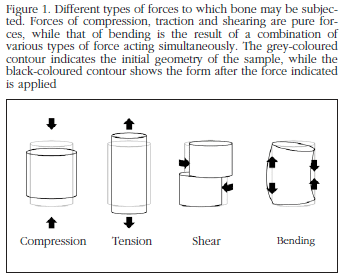The displacement (δ) experienced by the body or structure on which a force is exerted is proportional to its magnitude within the elastic limit, but this proportionality is not the same for all cases or for all directions. The mechanical characteristics of a material are measured in a test machine which subjects the object to a force of known magnitude and measures changes in its dimensions. When a mechanical test is carried out on an object a load-displacement curve is obtained which defines the total strain of the object in the direction of the application of the force. The load-displacement curve is used to measure the strength and rigidity of the structure, however, to compare different materials, standardisation by means of a stress-strain curve is necessary. The load and displacement may be normalised respectively as stress and strain using the dimensions of the object  (Figure 2).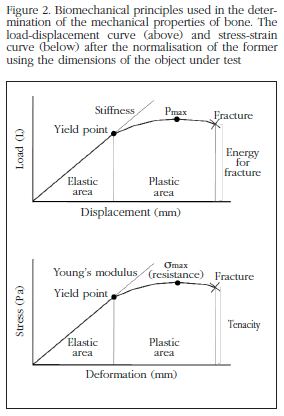Stress and strain

Stress (σ) is the internal resistance of an object to a force acting upon it, and is measured in pascals (Pa), 1 Pa being a force of 1 N distributed over an area of 1 m2. In the case of bone, the physiological values of interest are found in the interval of millions of pascals (megapascals MPa) . Strain (ε) is another concept necessary in describing the mechanical behaviour of materials and represents the changes in the dimensions of an object subject to the action of a force. Strain may be expressed in units of absolute length or in units of normalised length ε = ΔL/L, where ΔL is the variation in length and L is the initial length, which means that in this case it is a non-dimensional magnitude (mm/mm). Strain is also usually expressed as a percentage.

On occasions, in subjecting a body to the action of a force, the body is capable of returning all the energy used in its deformation once this force ceases (elastic behaviour). However, in certain circumstances this is not possible, the strain suffered by the body being irreversible (plastic behaviour). If we subject a bone to the progressive action of a force, the two types of strain are produced successively (Figure 2), by which the bone is said to have an elastic-plastic behaviour. From the stress-strain curve we may obtain a great quantity of information on the properties of the material. It is possible to distinguish between a first area in which the stress and the strain are proportional (the linear area of the curve, which corresponds to the elastic area, in which Hooke's law of elasticity applies) and another area in which the original shape of the body does not recover even when the load ceases to be applied (the plastic zone or zone of irreversible strain). The point of transition between the elastic area and the plastic area is called the yield point, which corresponds to the yield strain (εy) and to the yield stress or maximum elastic resistance (σy), which estimates the capacity of a material to become strained without suffering microfractures. In a certain area of the plastic region there is a point corresponding to the maximum stress (ultimate stress, σult), from which microfractures occur which are responsible for the fact that even with a reduction in stress the deformation of the sample increases.

Mechanical properties of materials

The mechanical properties of a material are all those characteristics which allow it to be differentiated from others from the point of view of its mechanical behaviour.

Elasticity and plasticity

Elasticity is the property of a material to recover its initial form once a force has ceased to be a applied. Plasticity is the opposite property: plastic strain is maintained even when the force ceases. The proportions of total resistance borne in conditions of elastic and plastic behaviour may be expressed in the following way:

Elasticity = σult- σy

Plasticity = (σult - σy)/σult

An example of elastic material is rubber, while a plastic material would be, for example, plasticine.

Stiffness and flexibility

Stiffness is a characteristic of materials for which a major force is necessary to induce a small elastic strain in the material. It corresponds to the slope of the elastic area of the load-displacement curve (extrinsic stiffness, S), expressed as N/m; of the stress-strain curve (elasticity or Young's modulus, E), expressed as Pa. When speaking of stiffness it should be as a characteristic of the whole structure, while the stiffness of a material is indicated by Young's modulus. The concept of stiffness is commonly to be found in both contexts, which may give rise to confusion, which is why it is recommended that flexibility be used to describe the structural characteristic and modulus of elasticity for property of a material . Flexibility is the property which is the opposite to stiffness. A flexible material is that which shows great strain in the elastic zone before getting to the plastic zone. A material with a low Young's modulus will undergo great strain with little stress, while a material with a high Young's modulus will suffer small strains with large stresses. Paper or cloth for example are flexible materials. On the other hand, ceramics or glass are stiff materials, since when they are bent they break.

Tenacity, work to break and resilience

Toughness (or tenacity, u) is the capacity of a material to resist plastic strain. Toughness represents the quantity of energy absorbed up to the point of the appearance of a fracture . It is obtained quantitatively by calculating the area under the curve which forms the elastic and plastic sections of a stress-strain graph. Those materials which, like iron, resist blows without breaking are called tough. If the information on toughness is found in the load displacement curve, which is to say that it refers to the structure and not the material, one speaks of the energy necessary for fracture, or work to break (U).

Toughness should not be confused with surface hardness, which refers to the resistance of a material to be scratched or dented. Toughness takes account of the energy absorbed up until the fracture happens, being calculated by means of the area below the curve from the initial point up to the breaking point, while resilience represents the energy which the material is able to absorb without experiencing permanent strain, which is to say it only takes into account the quantity of energy absorbed during the elastic strain (which would correspond to the area below the curve from the initial point to the yield point). Resilience is defined as the capacity of the material to resist elastic strain. A high degree of resilience is found, for example, in the cartilage of the joints.

Strength

Therefore the characteristics obtained from the load-displacement curve (maximum force, maximum displacement, extrinsic stiffness and work to break) will provide us with information relating to the extrinsic or structural properties, referring to the bone as structure. However, the information which we obtain from the stress-strain curve (maximum stress, maximum strain, Young's modulus and toughness) refer to the bone tissue as material, being known as intrinsic or material biomechanical properties. But what then is bone strength? Strength estimates the effective opposition of a material to losing its integrity, which is to say, of being fractured, being defined as the force necessary to trigger the mechanical break of the material under specific load conditions. From the yield point in the stress-strain graph starts what is called the plastic zone, in which small increments in stress provoke relatively large increases in strain/deformation, which indicate that a part of the structure has begun to break. Maximum strength, or simply, strength, is no more than the maximum stress necessary to fracture the material. The maximum force is used also on occasions as an indicators of the strength of an object, but it is necessary to take into account that this should only be used to compare samples of the same composition and size.

Concepts referring to the dimensions of the material

Poisson coefficient

If an object is subjected to a uniaxial compression force its dimensions reduce in the direction of the force (longitudinal direction) and increase in the transverse direction. If, on the other hand, we apply to the object a force of traction or tension, the dimensions of the object reduce in the transverse, and increase in the longitudinal, direction (Figure 1). The relationship between the strains in the two directions is given by the Poisson ratio, ν, such that: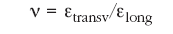Moment of inertia

Inertia is the property of an object to resist a change in its movement and is described in Newton's first law of motion ("Any body will continue in a state of rest or uniform and rectilinear motion unless obliged to change this state due to forces acting on it). Any object which spins on an axis develops rotation inertia, which is to say, a resistance to change in velocity or in its axis of spin. The inertia of a rotating object is determined by its moment of inertia, (I), which is the resistance with which a rotating body opposes a change to its spin velocity. Considering a large bone to be a cylinder of bone, the moment of inertia of the elliptical transverse section may be calculated as [10,13-15].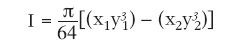where x1 is the greatest external diameter of the transverse section at the point of application of the force, and y1 is the smallest external diameter, x2 the greatest internal diameter and y2 the smallest internal diameter (Figure 3).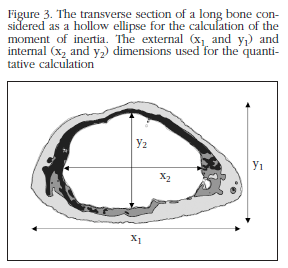Inertia may be interpreted as an analogue of mass in uniform rectilinear movement. The moment of inertia would reflect, therefore, the distribution of the mass of a body with respect to its axis of spin. The moment of inertia depends exclusively on the geometry of the body and the position of the axis of spin (and not on the forces which intervene in the movement), in such a way that the greater the distance between the mass and the centre of spin, the greater its value.

Viscoelasticity

While the mechanical behaviour of many solids approximates Hooke's law (elastic behaviour) and that of many liquids to Newton's law (viscous behaviour), both laws are idealisations. In applying a load on an elastic solid this is strained until the force ceases and the strain returns to its initial value. If the load is applied to a viscous fluid it is also strained, but does not recover once the load ceases. In the case of a viscoelastic material the object to which the force is applied recovers part of the strain. Viscoelasticity is a phenomenon which describes the mechanical characteristics of materials as a function of time. Bone, as with most biological materials, is a viscoelastic material. To quantify the mechanical properties of a viscoelastic material we need to take into account stress relaxation and creep. Stress relaxation is the reduction in tension in a material subject to a constant strain, while creep is the gradual increase in the strain of a material subject to a constant load .

Viscoelastic behaviour is described using three variables: storage modulus, E', loss modulus, E" and loss tangent, tan δ). In viscoelastic materials the complex modulus, E* is calculated, which is a measure of the resistance with which the material opposes the strain and combines the elastic response, through the storage modulus (related to the storage of energy), and viscosity, through the loss modulus (related to the dissipation of energy).

Fracture and fatigue

As has already been seen, when a force is exerted on bone a deformation will occur in elastic conditions first and in plastic conditions later, until the point in time at which a fracture or mechanical break of the bone occurs. However, fractures commonly appear in bone without it having reached the maximum stress which it can endure. Fatigue is the damage which occurs in a material due to repeated stress below the maximum level. The cycles of load on a material may provoke a failure even though these loads are below the value for rupture. For example, in a human bone a stress may provoke a microfracture without the bone breaking completely. If this stress is repeated over a number of consecutive cycles, the microfracture will propagate, causing total rupture of the structure.

Mechanical trials of compression and traction

Mechanical trials of compression and traction are standardised tests in which a sample is subjected to a uniaxial force in a universal machine for controlled force or displacement tests (Figure 5).

The specimens for traction or tension trials need to be cylindrical or prismatic in shape, with widened ends, both to facilitate their subjection to the test machine, and to ensure their rotation in within the area of smallest section (Figure 4). Although the traction trial is one of the most precise methods to determine the mechanical properties of bone, the obtaining of bone samples for these trials is highly complex. In the case of trabecular bone samples, which may fracture easily when subjected to the instruments of the test machine, the ends of the samples are usually coated in plastic resin. The proportions of the different measures of the specimen are derived from the ASTM American Society for Testing and Materials) standards.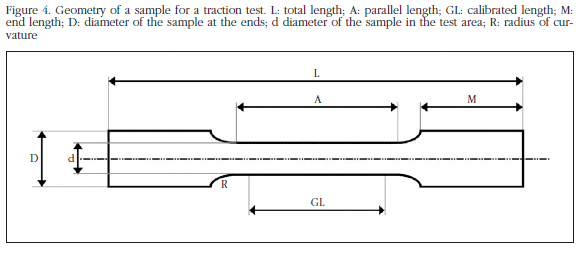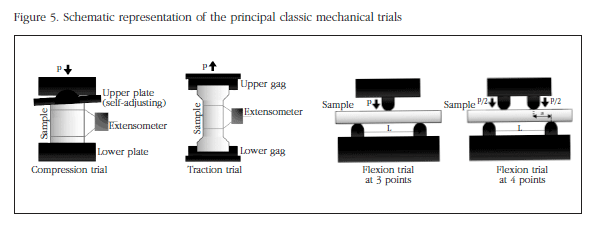In the case of compression trails, the samples commonly consist of 8 mm cubes or cylinders of 8 mm in diameter. In compression trials of trabecular bone it has been shown that the Young modulus is found to be low due to the effects of friction between the plates and the surfaces of the sample, and the damage done to those surfaces while the sample is being obtained . To minimise these effects cylindrical samples are recommended with a length-diameter ratio of 2:1 . It is very important to ensure that the end surfaces are parallel to each other to avoid errors during the test. There are now even autoadjustable compression plates available to compensate for a lack of alignment of the surfaces.

Both in the compression trials and those of traction, the use of en extensometer is normal, which can be set to the measurements of the object to be tested. This fact, in addition to allowing the determination of the deformation occurring in the specimen, reduces possible errors in the measurement by excluding deformation caused by the grips, the plates of the machine, etc. The sample is subjected to compression or traction at a constant force (N/s) or displacement (m/s) and the data relating to the force and shortening or lengthening of the sample are gathered by means of a force transductor and an extensometer.

The stress may be calculated as: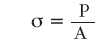where P is the load applied, and A the area of transverse section of the sample. The strain is calculated as:where δ is the displacement of the sample and L0 its initial length. Hence we can obtain a stress-strain curve. From this curve we can calculate Young's module as the incline of the linear region of the curve (elastic zone).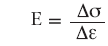The area under the stress-strain curve gives us the value of toughness of the material (u). The value of maximum stress (σult) will give us the strength of the bone under force of traction or compression.

Mechanical trials of torsion

Trials of torsion are carried out to determine the mechanical properties of an object when a shear stress is applied. The samples for torsion trials (normally with a circular transverse section) are fixed at the ends to the test machine supports and twisted in opposite directions from their ends, producing shear stress until the sample snaps . The torque, T, is measured by means of a transductor and the twist angle, Φ, using a sensor, both incorporated into the test machine. With these two variables and the dimensions of the sample being tested, we can calculate the shear stress, τ:where T is the torque, r the radius of the sample, Ip the polar moment of inertia of the transverse section. The shear strain, γ, will be: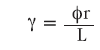where Φ is the twist angle, r the radius of the sample and L its length.

The shear modulus, G, is obtained from the incline of elastic region of the curve: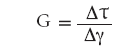As with the compression or traction tests, the maximum stress (τult) indicates to us the resistance of bone to torsion.

Mechanical tests of bending

There are two normal types of bending tests: bending at three points and bending at four points (Figure 5). In both cases the sample is fixed on two supports, but for the bending at three points the force is applied on the upper part in the centre of the specimen (being applied at the centre, the maximum moment of bending), while for bending at four points, two equal forces are applied symmetrically on the upper side, in such a way that the moment of bending is spread uniformly in the area between the two points of application . These tests are often used to determine the strength of long bones. Due to the fact that it is relatively simple to obtain samples, it is used extensively. When a bone is loaded while bending it is subject to a combination of compression (which act on one side of the bone) and traction forces (which act on the opposite side). As bone is less resistant to traction, the fracture is initiated on the surface which undergoes traction forces, propagating towards the compression surface and provoking the appearance of shearing forces, until a "butterfly wing" fracture occurs (with two oblique lines of fracture which form an angle between them and delimit a triangular fragment), characteristic of the two bending tests.

Using the theory of beam bending and assuming that bone has an elastic linear behaviour, we calculate the stress and strain in a flexion test at three points in the following manner [10,16,20]: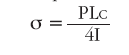where P is the load applied, L is the distance between the supports, c is half the lowest external diameter of the transverse section of the bone at the point of application of the force (mid section of the bone diaphysis), and I the moment of inertia of the elliptic transverse section. The moment of inertia for a hollow ellipse may be calculated in the way we have seen in the section "Concepts referring to the dimensions of the material". The strain will be obtained thus: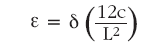An estimate of the elasticity modulus may be calculated from the load-displacement curve obtained , calculating the moment of inertia (I) and with the value of the distance between supports L, as: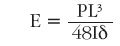Similarly, for the bending tests at four points, we calculate the stress as:where a is the distance between one support and the nearest point of application of the force. The elasticity modulus is estimated with the following formula :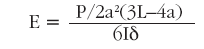Conclusions

The biomechanical behaviour of bone is extremely complex due to its heterogeneous, anisotropic and viscoelastic character. In this work the basic concepts of material mechanics, as well as certain properties characteristic of bone, are presented. These are all necessary in characterising this behaviour, whose understanding is important in interpreting the great quantity of information which we may find in the literature referring to the mechanical properties of bone.

In a mechanical trial the relationship between the force which we apply to a body and the displacement it undergoes is studied. The relationship between force and displacement is known as stiffness, while the maximum work carried out by the force in deforming the body is known as work to break. All these concepts are used frequently in mechanical tests with samples of bone. However, it should be taken in to account that these parameters describe an effect on which the structure of bone has a great influence. Thus, force, displacement, stiffness and work to break are known as extrinsic or structural properties. Let us imagine a cylinder of titanium and a cylinder of wood of the same diameter. The cylinder of titanium will be capable of resisting much greater forces than the cylinder of wood, since it is a stronger material. However, if we carry out a mechanical test on a piece of titanium wire and a very thick log of wood, the latter will withstand greater forces, which is not to say that from a material perspective wood is stronger than titanium. For this reason it is necessary to eliminate the contribution of the geometry of the sample to the biomechanical measures, calculating the intrinsic or material biomechanical properties of the body under test. This is done by normalising the force applied by dividing it between the area to which it is applied, obtaining the stress, and dividing the displacement by the initial length of the body, obtaining the strain. The relationship between them will give use the elasticity modulus and the area under the curve will indicate the toughness of the material. Due to the anisotropic character of bone, its biomechanical properties vary as a function of the direction in which the force is applied. Thus, the bone will show different strength depending on whether forces of compression, traction or shearing are applied. Compression tests are often used for trabecular or cortical bone samples, or for vertebral bodies. The long bones such as the femur or tibia are usually subjected to traction, torsion or bending tests. In these, there is a combination of compression forces on the side to which the force is applied and of traction forces on the opposite side.

The relationship between structural properties, material properties and the mechanical behaviour of bone is complicated and this is a challenge. An understanding of this relationship is of great importance, since it helps us to understand of the behaviour of bone subjected to constant physiological loads, identifies the areas most susceptible to fracture and allows the prediction of different pathologies in relation to bone strength, and their treatment. In a second part to this work we analyse the hierarchical structure of bone and the biomechanical tests which are carried out nowadays in different areas, as well as alternative techniques to the classic biomechanical trials for the determination of bone strength.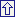Correspondence:
David Guede
Trabeculae, S.L.
Parque Tecnolóxico de Galicia,
Edificio "Tecnópole I" Local 22
32900 San Cibrao das Viñas - Ourense (Spain)
e-mail: dguede@trabeculae.com

Bibliography

1. Frost HM. Bone "mass" and the "mechanostat". A proposal. Anat Rec 1987;219:1-9.         [ Links ]

2. Martin RB. Determinants of the mechanical properties of bone. J Biomech 1991;24(S1):79-88.         [ Links ]

3. Ferretti JL. Biomechanical properties of bone. En: Genant HK, Guglielmi G, Jergas M, editors. Bone densitometry and osteoporosis. Springer (Berlin, Germany) 1998;pp.143-61.         [ Links ]

4. Faulkner KG, Cummings SR, Black D, Palermo L, Glüer CC, Genant HK. Simple measurement of femoral geometry predicts hip fracture: The study of osteoporotic fractures. J Bone Miner Res 1993;8:1211-7.         [ Links ]

5. Millard J, Augat P, Link TM, Kothari M, Newitt DC, Genant HK, et al. Power spectral analysis of vertebral trabecular bone structure from radiographs: Orientation dependence and correlation with bone mineral density and mechanical properties. Calcif Tissue Int 1998;63:482-9.         [ Links ]

6. Lespessailles E, Jullien A, Eynard E, Harba R, Jacquet G, Ildefonse JP, et al. Biomechanical properties of human os calcanei: Relationships with bone density and fractal evaluation of bone microarchitecture. J Biomech 1998;31:817-24.         [ Links ]

7. Majumdar S, Lin J, Link T, Millard J, Augat P, Ouyang X, et al. Fractal analysis of radiographs: Assessment of trabecular bone structure and prediction of elastic modulus and strength. Med Phys 1999;26:1330-40.         [ Links ]

8. Prouteau S, Ducher G, Nanyan P, Lemineur G, Benhamou L, Courteix D. Fractal analysis of bone texture: A screening tool for stress fracture risk? Eur J Clin Invest 2004:34:137-42.         [ Links ]

9. An YH, Barfield WR, Draughn RA. Basic concepts of mechanical property measurement and bone biomechanics. En: An YH, Draughn RA, editors. Mechanical testing of bone and the bone-implant interface. CRC Press LLC (Boca Raton, FL, USA) 2000;pp.23-40.         [ Links ]

10. Turner CH, Burr DB. Basic biomechanical measurements of bone: A tutorial. Bone 1993;14:595-608.         [ Links ]

11. Currey JD. Bone strength: What are we trying to measure? Calcif Tissue Int 2001;68:205-10.         [ Links ]

12. Ritchie RO, Koester KJ, Ionova S, Yaoc W, Lane NE, Ager III JW. Measurement of the toughness of bone: A tutorial with special reference to small animal studies. Bone 2008;43:798-812.         [ Links ]

13. Jämsä T, Jalovaara P, Peng Z, Väänänen HK, Tuukkanen J. Comparison of threepoint bending test and peripheral quantitative computed tomography analysis in the evaluation of the strength of mouse femur and tibia. Bone 1998;23:155-61.         [ Links ]

14. Turner CH. Biomechanics of bone: Determinants of skeletal fragility and bone quality. Osteoporos Int 2002;13:97-104.         [ Links ]

15. Saffar KP, Jamil Pour N, Rajaai SM. How does the bone shaft geometry affect its bending properties? Am J Appl Sci 2009;6:463-70.         [ Links ]

16. Wang S, Nyman JS, Dong X, Leng H, Reyes M. Current mechanical test methodologies. En: Athanasiou KA, editor. Fundamental biomechanics in bone tissue engineering. Morgan & Claypool Publishers (Lexingyon, KY, USA) 2010;pp.43-74.         [ Links ]

17. Linde F, Hvid I, Madsen F. The effect of specimen size and geometry on the mechanical behavior of trabecular bone. J Biomech 1992;25:359-68.         [ Links ]

18. Keaveny TM, Borchers RE, Gibson LJ, Hayes WC. Theoretical analysis of the experimental artifact in trabecular bone compressive modulus. J Biomech 1993;26:599-607.         [ Links ]

19. ANSI/ASAE S459 MAR98, approved Feb 1993; reaffirmed Mar 1998 by American National Standards Institute. Shear and three-point bending test of animal bone.         [ Links ]

20. Lopez MJ, Markel MD. Bending tests of bone. En: An YH, Draughn RA, editors. Mechanical testing of bone and the bone-implant interface. CRC Press LLC (Boca Raton, FL, USA) 2000;pp.207-17.         [ Links ]

21. Sharir A, Barak MM, Shahar R. Whole bone mechanics and mechanical testing. Vet J 2008;177:8-17.         [ Links ]Todo el contenido de esta revista, excepto dónde está identificado, está bajo una Licencia Creative Commons